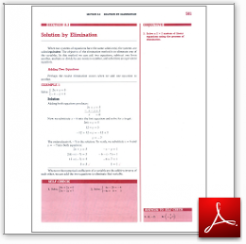Algebra Math Book - Introductory Algebra Thank you for your continual support.

 The power of mathematics rests in the logic of thinking.ID: Sec8-3
Description: Adding Two Equations-Subtracting Two Equations-Multiply by Constant-Equations with Infinitely Many Solutions-No Solution
Price: 1.99

# Algebra Math book - Introductory Algebra - Chapter 8 - Section 3 - Solution by Elimination

## Section 8.3 - Objectives

3.  Solve a 2 x 2 system of linear equations using the substitution method.

Navigate to
Previous Section or Next Section
or Chapter 8 Details or Other Chapters

This section of my algebra math book, Introductory Algebra, also includes in the download:

• Cover Sheet